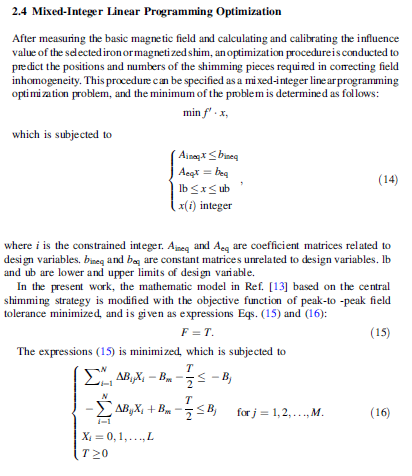# Zero result for mixed integer optimisation

Hello everybody,
I am trying to solve a mixed integer optimisation problem using mosek in CVX.
Here is the problem I am trying to solve. I want to minimise T

and here is the syntax and the result after optimisation.
Have I done any error?
Any help would be appreciated!

We don’t have your input data, and therefore can not know whether you have set up the problem correctly. But CVX reports that the problem is solved, with cvx_optval and therefore T = 0. Given the constraint T >= 0, if T = 0 (with the reported optimal values of the other variables) is feasible, which CVX reports it to be, it must be optimal. If you disagree with this answer, then there is a problem with your input data or model (likely), or the optimal variable values (argmin) returned by CVX do not satisfy one or more of your inequality constraints within tolerance, which would be indicative of an extreme numerical sensitivity or a bug in CVX and/or MOSEK (less likely, unless you are using CVX 3.0beta, in which case bug is not so unlikely).

Thank you for your reply. Even when the constraint T>0 the optimal value stays the same T = 0. This is what I am trying to understand!

http://cvxr.com/cvx/doc/dcp.html#strict-inequalities

I agree with Mark, you probably need to think more about what your model really is trying to achieve.

Thank you so much for your answer and the the link above. From the link above I could understand that cvx doesn’t differentiate between “>=” and “>” so that “=” is always involved! right? .
What the model is trying to achieve is this: the values of Xi (that is the position) when T is minimised. This is the goal of my optimisation in one sentence.
This work was done before according to different publications. This is the model in the publication I rely on:where T, Xi and Bm are unknown variables.
Did I write the objective function correctly?

It looks like you’re missing a lower bound of zero on position. Presuming `L` in (16) is 3, per your upper bound on position, perhaps you should change that to
`0 <= position <= 3`

Does your optimal position have any negative elements? If so, adding the lower bound of zero to position might be the (a) "fix: you need.

Of course we have no way of knowing whether your other inputs are correct.

I did change the lower bound of the position, however,that’s made no difference.
Xi represents the number of pieces at position i , therefore, the optimal position values cannot be negative at all.